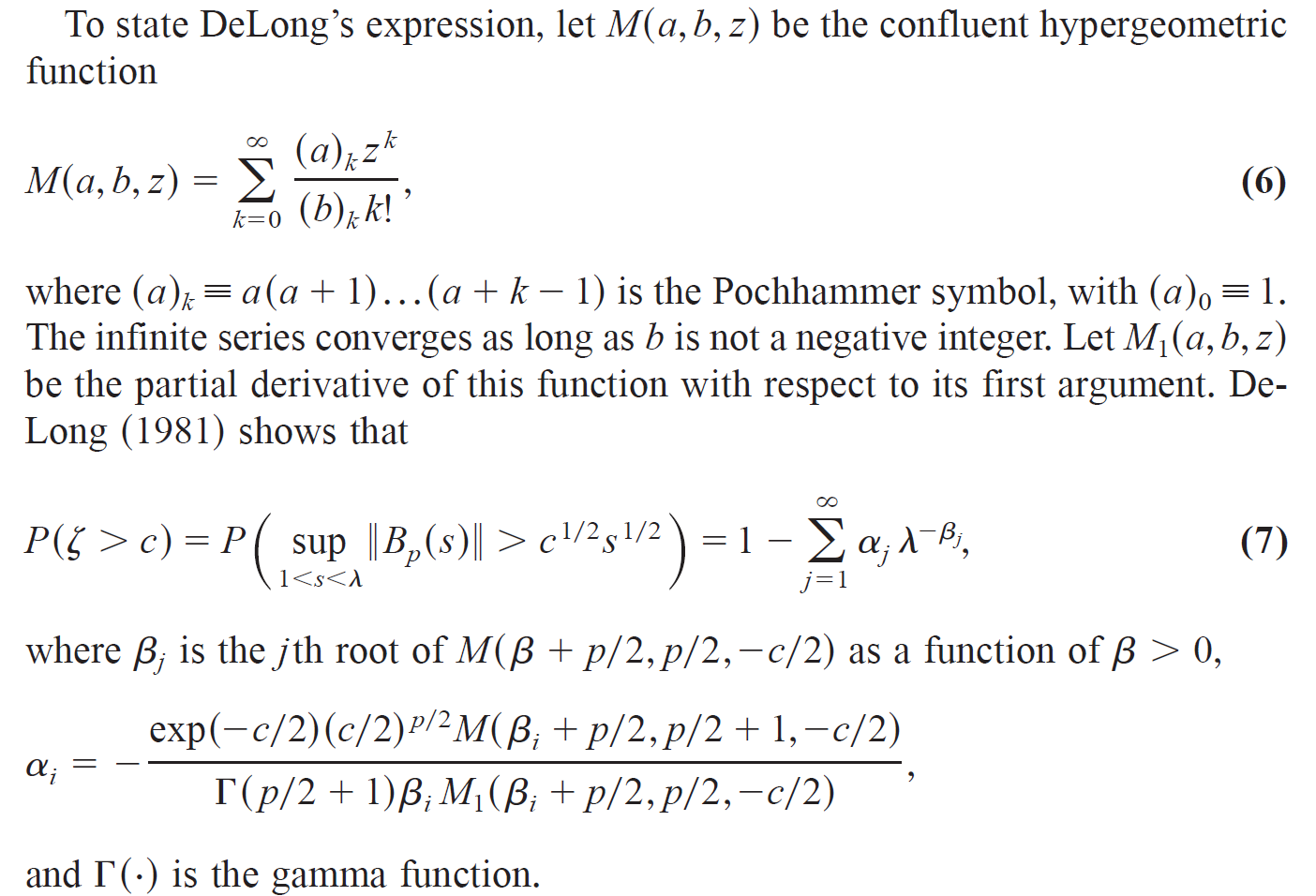Exact p Values for Unknown Structural Breakpoint Tests

p Value Calculator
A virtual calculator that computes exact p values for structural break tests when the breakpoint is unknown may be downloaded by clicking on the link above. The calculator uses the algorithm described in Estrella (2003). After installation in Windows, running the program opens a window that asks for the number of parameters (p), the proportion of the sample excluded from each end (π0) and the value of the supremum statistic (c), all as defined in Andrews (1993) and Estrella (2003). The program then returns the corresponding p value and prompts for an optional new iteration.

Computation
Andrews (1993) showed that the p values of a class of statistical tests for an unknown structural breakpoint may be obtained from the probability distribution of a Bessel process, and referred to DeLong (1981) for an expression that represents values of that distribution as an infinite sum. Estrella (2003) proposed an efficient algorithm to compute exact numerical values of that sum. Specifically, define the following terms.

p = number of parameters that are allowed to change
c = supremum of test statistic (Wald, Lagrange multiplier or likelihood ratio)
π0 = proportion of sample not tested at each end (0 < π0 < 1/2)
λ = ( (1 - π0) / π0 ) ^ 2

The p value of the structural break test is obtained by evaluating the probability in equation (7) of the following excerpt from Estrella (2003). The article gives a detailed description of the algorithm, which searches recursively for roots in unit intervals along the real line and computes a root numerically when one is detected in an interval. A form of the algorithm was first developed in joint work with Fuhrer (2003).Development
Estrella, Arturo (2003) “Critical values and p values of Bessel process distributions: Computation and application to structural break tests.” Econometric Theory 19, 1128-1143.

Estrella, Arturo and Jeffrey C. Fuhrer (2003) “Monetary policy shifts and the stability of monetary policy models.” Review of Economics and Statistics 85, 94-104.

Background
D.W.K. Andrews (1993) "Tests for parameter instability and structural change with unknown change point." Econometrica 61, 821–856.

David M. DeLong (1981) "Crossing probabilities for a square root boundary by a Bessel process." Communications in Statistics—Theory and Methods A10, 2197–2213.

Illustrative Applications
Estrella, Arturo, Anthony P. Rodrigues and Sebastian Schich (2003) “How stable is the predictive power of the yield curve? Evidence from Germany andthe United States.” Review of Economics and Statistics.

Omtzigt, Pieter and Paolo Paruolo (2005) “Impact factors.” Journal of Econometrics.

Rosa, Carlo (2011) “Words that shake traders: The stock market's reaction to central bank communication in real time.” Journal of Empirical Finance.

Ng, Eric (2012) “Forecasting US recessions with various risk factors and dynamic probit models.” Journal of Macroeconomics.

Chen, Sanpan, Guowei Cui and Jianhua Zhang (2017) “On testing for structural break of coefficients in factor-augmented regression models.” Economics Letters.

Hidalgo, Javier and Marcia Schafgans (2017) “Inference and testing breaks in large dynamic panels with strong cross sectional dependence.” Journal of Econometrics.